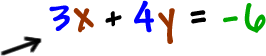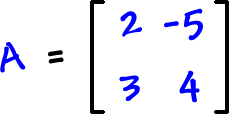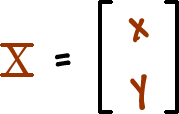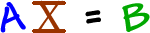So, what's all this fuss about inverse matrices?

You can use them to solve systems of equations.

Check it out:Let's get that coefficient matrix:Then, we'll need matrices forour variables our "= guys"

In short, this system can be rewritten asand we can use algebra to solve it!